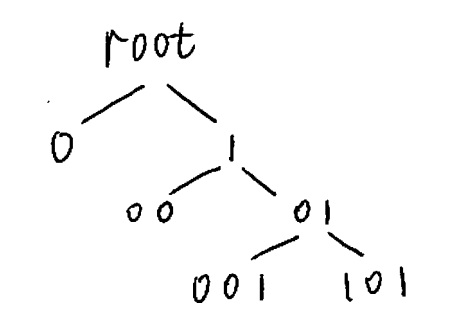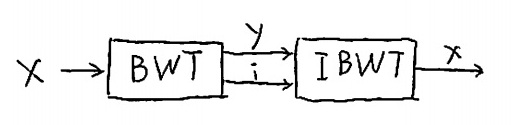# 0.4 Universal compression for context tree sources  (Page 2/3)

 Page 2 / 3

We note in past that there is no need to spend a bit to encode leaves of depth $D$ . To see this, consider a procedure for encoding the structure of a tree:

Consider the tree sourced depicted in [link] . In order to encode the structure of this tree, we will utilize the followingprocedure. (Such a procedure has appeared, for example, in  [link] .)Tree used in [link] to demonstrate how the structure of the source is encoded.

Start from root. [procedure(root)]
1. If node $S$ is of depth $D$ (maximum), then return.
2. If node $S$ is internal node, then {
encode 0
procedure(0S)
procedure(1S)
} else encode 1.
3. return.

Let us now simulate the procedure, the procedure will traverse through the following states of the tree in [link] while outputting the corresponding bits.

 Source root 0 1 01 001 101 11 Encoded symbol 0 1 0 0 1

Returning to tree pruning, following [link] we see that we must initialize $\text{MDL}\left(s\right)=\text{KT}\left({n}_{x}\left(s,0\right),{n}_{x}\left(s,1\right)\right)$ for $s$ of full depth $|s|=D$ without the extra bit.

At the end of the pruning procedure, ${T}_{\left\{\right\}}^{*}$ the maximizing tree for the root, will be the optimal tree for universal coding.

## Burrows wheeler transform

The Burrows Wheeler transform (BWT) was proposed by Burrows and Wheeler in 1994  [link] (see also the analysis by Effros et al.  [link] and references therein). It is an invertible permutation sort that sorts symbols according to their contexts. Thatway, the symbols that were generated by the same state of the context tree are grouped together, which as we will see is advantageous.

To compute the BWT, we first compute all cyclical shifts of the input $x$ . Next, we sort the cyclical shifts.The output of the BWT consists of $y$ , the last column of the matrix of sorted shifts, and $i$ the index of the original version. We illustrate with an example.

Consider the input $x=banana$ . First, we compute the cyclic shifts and their sorts.

 All Shifts Sorted banana abanan abanan anaban nabana ananab anaban banana nanaba nabana ananab nanaba

The output of the BWT consists of $y=nnbaaa$ , the last column of the matrix of sorted shifts (to the right), and the index $i=4$ containing the original input.

Interestingly, we can recover $x$ from $y$ and $i$ . Seeing that $y$ is structured and thus quite compressible, the BWT can be used as a compression system; a building block that illustrates such a system appearsin [link] .Typical compression system using the Burrows Wheeler transform  [link] .

To see that the BWT is invertible, let us work out how to do this by continuing our example.

In the matrix of sorted shifts, column 1 is a sorted version of column  $n$ , which we know.

 Column 1 Column n a n a n a b b a n a n a

Now take column  $n$ and put it before column 1:

 Column n Column 1 n a n a b a a b a n a n

We now sort these rows, which each consist of 2 symbols: $ab$ , $an$ , $an$ , $ba$ , $na$ , and $na$ . Now fill column 2 of the sorted shifts matrix accordingly.

 Columns 1–2 Column  $n$ ab n an n an b ba a na a na a

The entire matrix can be unraveled, and the row containing the original $x$ is indexed by $i$ .

What is the BWT good for? The key property of the BWT is that symbols generated by the same state are grouped together in $y$ . To see this, note how the last column  $n$ can be rotated to a position to the left of column 1, and symbols that came before the same prefix appear together.(To bunch together symbols generated by the same suffix, we can reverse the order of symbols in $x$ before running the BWT.) Therefore, $y$ has the form of a piecewise i.i.d. sequence  [link] , where segments generated by the same state of the context tree are bunched together.

#### Questions & Answers

Is there any normative that regulates the use of silver nanoparticles?
what king of growth are you checking .?
Renato
What fields keep nano created devices from performing or assimulating ? Magnetic fields ? Are do they assimilate ?
why we need to study biomolecules, molecular biology in nanotechnology?
?
Kyle
yes I'm doing my masters in nanotechnology, we are being studying all these domains as well..
why?
what school?
Kyle
biomolecules are e building blocks of every organics and inorganic materials.
Joe
anyone know any internet site where one can find nanotechnology papers?
research.net
kanaga
sciencedirect big data base
Ernesto
Introduction about quantum dots in nanotechnology
what does nano mean?
nano basically means 10^(-9). nanometer is a unit to measure length.
Bharti
do you think it's worthwhile in the long term to study the effects and possibilities of nanotechnology on viral treatment?
absolutely yes
Daniel
how to know photocatalytic properties of tio2 nanoparticles...what to do now
it is a goid question and i want to know the answer as well
Maciej
characteristics of micro business
Abigail
for teaching engĺish at school how nano technology help us
Anassong
Do somebody tell me a best nano engineering book for beginners?
there is no specific books for beginners but there is book called principle of nanotechnology
NANO
what is fullerene does it is used to make bukky balls
are you nano engineer ?
s.
fullerene is a bucky ball aka Carbon 60 molecule. It was name by the architect Fuller. He design the geodesic dome. it resembles a soccer ball.
Tarell
what is the actual application of fullerenes nowadays?
Damian
That is a great question Damian. best way to answer that question is to Google it. there are hundreds of applications for buck minister fullerenes, from medical to aerospace. you can also find plenty of research papers that will give you great detail on the potential applications of fullerenes.
Tarell
what is the Synthesis, properties,and applications of carbon nano chemistry
Mostly, they use nano carbon for electronics and for materials to be strengthened.
Virgil
is Bucky paper clear?
CYNTHIA
carbon nanotubes has various application in fuel cells membrane, current research on cancer drug,and in electronics MEMS and NEMS etc
NANO
so some one know about replacing silicon atom with phosphorous in semiconductors device?
Yeah, it is a pain to say the least. You basically have to heat the substarte up to around 1000 degrees celcius then pass phosphene gas over top of it, which is explosive and toxic by the way, under very low pressure.
Harper
Do you know which machine is used to that process?
s.
how to fabricate graphene ink ?
for screen printed electrodes ?
SUYASH
What is lattice structure?
of graphene you mean?
Ebrahim
or in general
Ebrahim
in general
s.
Graphene has a hexagonal structure
tahir
On having this app for quite a bit time, Haven't realised there's a chat room in it.
Cied
what is biological synthesis of nanoparticles
how did you get the value of 2000N.What calculations are needed to arrive at it
Privacy Information Security Software Version 1.1a
Good
Got questions? Join the online conversation and get instant answers!By Anindyo MukhopadhyayBy Mariah HauptmanByBy Mistry BhaveshByBy